# Thermal Expansion and Contraction with Examples

Thermal Expansion and Contraction

Most of the matters, without some exceptions, expand with the increasing temperature. When you give heat to matters; speed of its particles increase and distance between them also increase which results in the increase of the volumes of matters. All expansions occurs in volume of the substance however, sometimes some of the dimensions of them expand more with respect to others. In this case we neglect the less expanded ones and assume expansion like linear expansion in long materials. Moreover, we take the expansion of plate as area expansion and finally we take the expansion in three dimensions as volume expansion.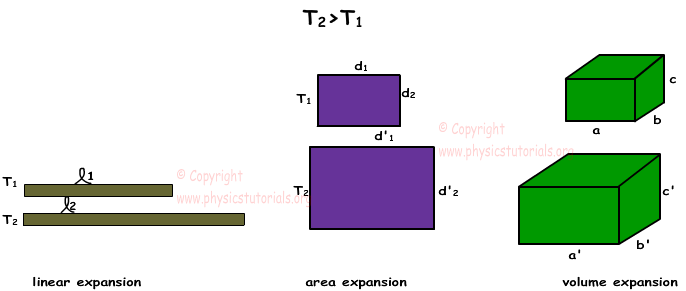Inverse of the expansion is called contraction, generally when matters lost heat and their temperatures decrease they contract. Now we will learn which factors effect expansion.

If the initial volumes, areas or lengths of the matters are big enough their expansions with the same temperature are also big. In other words, expansion or contraction is linearly proportional to the initial volume of the matter.

Different matters have different atomic structure, thus distances between the atoms are also different. They give different reactions to the same amount of temperature changes. So, another factor effecting expansion is type of matter.

Final factor that affects expansion is the amount of change in temperature. Larger the change in temperature results in larger the change in the volume of matter.

We get following formula from the explanations given above;

∆V=V0.α. ∆T

Where; V is the change in the volume, α is the coefficient of thermal expansion and ∆T is the change in the temperature of the matter.

α= Coefficient of thermal expansion is equal to the change in the volume of a unit of mass under 10C change in temperature.

Expansion in Solid Matters

We will examine this subject under three title, linear expansion, area expansion and volume expansion.

Linear Expansion: Picture given below shows the linear expansion of metal rod. When it is heated, its length increases.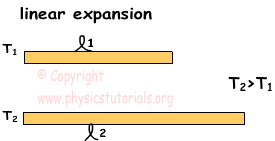Our formula for linear expansion is;

∆L=L0.α. ∆T

Where; ∆L is the amount of change in the length of the rod, L0 is the initial length of the road, α is the coefficient of linear expansion and ∆T is the change in the temperature of the matter.

Example: There are three same metal rods having same length and thickness. If the temperatures of them are given like; T, 2T and 3T find the relations of final lengths of the rods. (Rods are in contact)

We find the final temperatures of the system by the formula;

Tfinal=T1+T2+T3/3=6T/3=2T

Since the temperature of the first rod increase, its final length also increases. Temperature of the second rod stays same, thus there won’t be change in the length of this rod. Finally, temperature of the third rod decreases, thus its contract and final length of it decreases with respect to initial length. As a result relation of the final lengths of the rods;

L1>L2>L3

Area Expansion: When plate given below is heated, it expands in two dimensions X and Y. We find the area expansion with the given formula;

∆S=S0.2α. ∆T

Where; ∆S is the amount of change in the area of the plate, S0 is the initial area of the plate, 2α is the coefficient of area expansion and ∆T is the change in the temperature of the matter.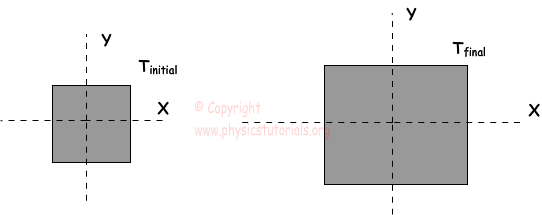Example: We cut a circular piece from the rectangular plate. Which ones of the processes given below can help us in passing through the circular piece from the hole?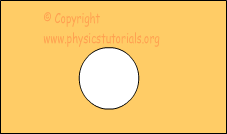I. Increasing the temperatures of rectangular plate and circular piece

II. Decreasing the temperature of the circular piece

III. Decreasing the temperatures of the rectangular plate and circular piece

I. If we increase the temperatures of the plate and circular piece, expansion of the hole and the circular piece will be the same. Thus, this option can help us.

II. If we decrease the temperature of the circular piece, it contracts and hole becomes larger than the piece. This option can also help us.

III. If we decrease the temperatures of the plate and circular piece,  hole and circular piece contract in same size. This process can also help us.

Volume Expansion: If the objects expand in volume with the gained heat, we call this volume expansion and find it with the following formula;

∆V=V0.3α. ∆T

Where; ∆V is the amount of change in the volume of the cube, V0 is the initial volume of the cube, 3α is the coefficient of volume expansion and ∆T is the change in the temperature of the matter.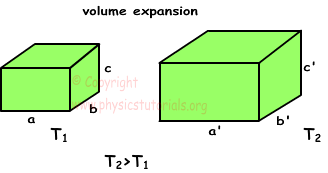Heat Temperature and Thermal Expansion Exams and Solutions

Related